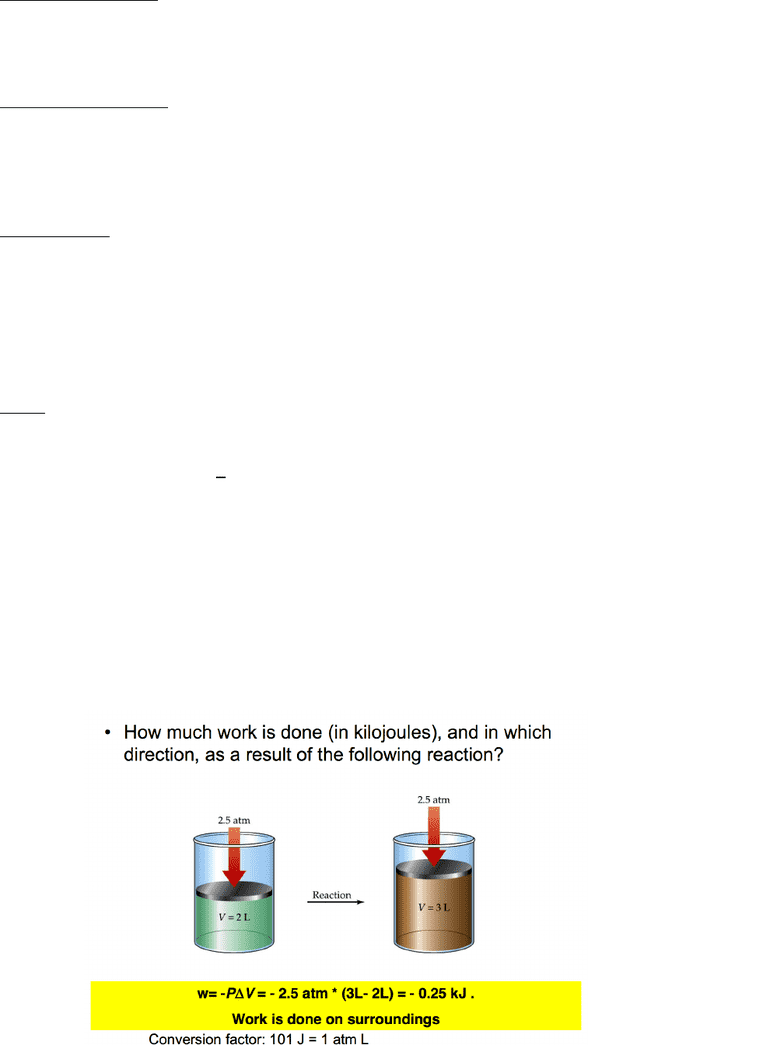# CHM139H1 Final: Thermochemistry Review

71 views13 pages
School
UTSG
Department
Chemistry
Course
CHM135H1
ProfessorThermochemistry: Chemical Energy:
Thermodynamics:
Deals with study of energy and its transformations (relative changes between initial
and finals)
What is energy?:
It is the capacity to do work or supply heat
Types of Energy: (units: J = kg2/s2); (1 calorie = 4.184 J)
1. Kinetic Energy:
a. Energy of motion
i. Mechanical, thermal and electrical energy
2. Potential Energy:
a. Stored energy
i. Gravitational, electrostatic and chemical potential energy
When energy is transferred from one object to another, it appears as work/heat
Each particle in a system has kinetic and potential energy (the sum of this gives us
the internal energy, E, of the system)
What is The System?:
It is the part of the world in which we have a special interest (all the rest are
surroundings)
1. Closed System:
I. Only energy can be gained or lost
2. Isolated System:
I. No matter or energy is exchanged
3. Open System:
I. Both energy and matter can be flow in and out
* we define the system as the object of interest. The surroundings are everything else*
* changes to the system and surroundings are EQUAL and OPPOSITE*
(if the system loses energy, surroundings must gain that energy)
The First Law of Thermodynamics: Law of Conservation of Energy:
Energy is conserved (cannot be created or destroyed), the total energy of the system
and surroundings are constant
Esystem + Esurrounding = constant
Unlock document

This preview shows pages 1-3 of the document.
Unlock all 13 pages and 3 million more documents.Thermodynamic Changes:
Change in internal energy:
o ΔE = EFinal - EInital
Energy is transferred as heat or work:
o ΔE = q + w
§ q = energy heat exchanged (negative if system heats surroundings)
§ w = energy transferred by doing work (negative if work is done by
surroundings)
Einital > Efinal
Einital < Efinal
H2 is pushing
piston, thus
doing work
on the system
Unlock document

This preview shows pages 1-3 of the document.
Unlock all 13 pages and 3 million more documents.Exothermic Reaction:
Energy is released ~ [reactants -> products + energy]
o System temperature decreases
o Surrounding temperature increases
Endothermic Reaction:
Energy is absorbed ~ [reactants + energy -> products]
o System temperature increases
o Surrounding temperature decreases
State Function:
Property than depends only the current state of the system (the path doesn’t matter)
o Only depends on initial and final state of the system
§ The change is zero if the final state is the same as original state
E and ΔE are both state function:
o q and w are not state functions
P, V and T are state functions
Work: (P-V work)
Pressure (P):
o Is the force (F) per unit area (A)
o 𝑃𝑟𝑒𝑠𝑠𝑢𝑟𝑒 = ' (
)
§ 1 N/m2 = 1 Pa
§ 1 atm = 101,325 Pa
Work (W):
o Is a force that produces an objects movement, times the distance moved (d)
o 𝑊𝑜𝑟𝑘 = 𝐹'𝑥'𝐷
§ 1 Nm = 1 J
§ 1 atm = 101 J
o Work = -PΔV
§ Negative because system does work on surroundings
Psystem = Psurroundings
Unlock document

This preview shows pages 1-3 of the document.
Unlock all 13 pages and 3 million more documents.

## Document Summary

Thermochemistry: chemical energy: deals with study of energy and its transformations (relative changes between initial and finals) It is the capacity to do work or supply heat. * we define the system as the object of interest. * changes to the system and surroundings are equal and opposite* (if the system loses energy, surroundings must gain that energy) The first law of thermodynamics: law of conservation of energy: energy is conserved (cannot be created or destroyed), the total energy of the system and surroundings are constant, esystem + esurrounding = constant. Thermodynamic changes: change in internal energy, energy is transferred as heat or work, e = q + w, e = efinal - einital. Q = energy heat exchanged (negative if system heats surroundings) W = energy transferred by doing work (negative if work is done by surroundings) H2 is pushing piston, thus doing work on the system.